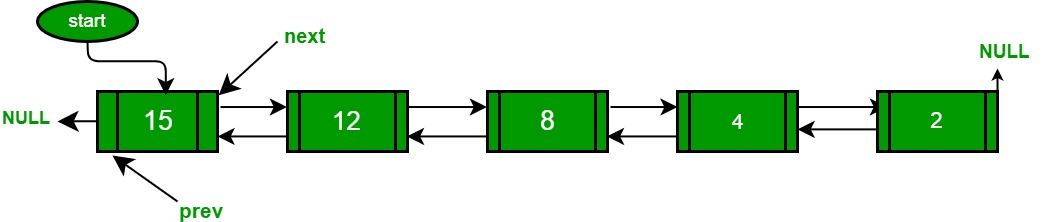GeeksforGeeks App
Open AppBrowser
Continue

# Python Program For QuickSort On Doubly Linked List

Following is a typical recursive implementation of QuickSort for arrays. The implementation uses last element as pivot.

## Python3

 `"""A typical recursive implementation of Quicksort for array """`` ` `""" This function takes last element as pivot,``   ``places the pivot element at its correct``   ``position in sorted array, and places all``   ``smaller (smaller than pivot) to left of``   ``pivot and all greater elements to right``   ``of pivot``"""` `"""`` ``i --> is the first index in the array`` ``x --> is the last index in the array`` ``tmp --> is a temporary variable for swapping values (integer)``"""``# array arr, integer l, integer h``def`  `partition (arr, l, h):``    ``x ``=` `arr[h]``    ``i ``=` `(l ``-` `1``)``    ``for` `j ``in` `range``(l, h):``        ``if` `(arr[j] <``=` `x):``            ``i ``+``=``1``            ``tmp ``=` `arr[i]``            ``arr[i] ``=` `arr[j]``            ``arr[j] ``=` `tmp` `    ``tmp ``=` `arr[i ``+` `1``]``    ``arr[i ``+` `1``] ``=` `arr[h]``    ``arr[h] ``=` `tmp``    ``return``(i ``+` `1``)` `"""``A --> Array to be sorted,``l --> Starting index,``h --> Ending index``"""` `# array A, integer l, integer h``def` `quickSort(A, l, h):``    ``if` `(l < h):``        ``p ``=` `partition(A, l, h) ``# pivot index``        ``quickSort(A, l, p ``-` `1``) ``# left``        ``quickSort(A, p ``+` `1``, h) ``# right` `# This code is contributed by humphreykibet.`

Can we use the same algorithm for Linked List?
Following is C++ implementation for the doubly linked list. The idea is simple, we first find out pointer to the last node. Once we have a pointer to the last node, we can recursively sort the linked list using pointers to first and last nodes of a linked list, similar to the above recursive function where we pass indexes of first and last array elements. The partition function for a linked list is also similar to partition for arrays. Instead of returning index of the pivot element, it returns a pointer to the pivot element. In the following implementation, quickSort() is just a wrapper function, the main recursive function is _quickSort() which is similar to quickSort() for array implementation.## Python3

 `# A Python program to sort a linked list using Quicksort``head ``=` `None` `# a node of the doubly linked list``class` `Node:``    ``def` `__init__(``self``, d):``        ``self``.data ``=` `d``        ``self``.``next` `=` `None``        ``self``.prev ``=` `None` `# A utility function to find last node of linked list``def` `lastNode(node):``    ``while``(node.``next` `!``=` `None``):``            ``node ``=` `node.``next``;``    ``return` `node;` `# Considers last element as pivot, places the pivot element at its``#   correct position in sorted array, and places all smaller (smaller than``#   pivot) to left of pivot and all greater elements to right of pivot``def` `partition(l, h):` `    ``# set pivot as h element``        ``x ``=` `h.data;``         ` `        ``# similar to i = l-1 for array implementation``        ``i ``=` `l.prev;``        ` `        ``j ``=` `l``        ` `        ``# Similar to "for (int j = l; j <= h- 1; j++)"``        ``while``(j !``=` `h):``            ``if``(j.data <``=` `x):``              ` `                ``# Similar to i++ for array``                ``i ``=` `l ``if``(i ``=``=` `None``) ``else` `i.``next``;` `                ``temp ``=` `i.data;``                ``i.data ``=` `j.data;``                ``j.data ``=` `temp;``            ``j ``=` `j.``next``                        ` `        ``i ``=` `l ``if` `(i ``=``=` `None``) ``else` `i.``next``;  ``# Similar to i++``        ``temp ``=` `i.data;``        ``i.data ``=` `h.data;``        ``h.data ``=` `temp;``        ``return` `i;` `# A recursive implementation of quicksort for linked list``def` `_quickSort(l,h):``    ``if``(h !``=` `None` `and` `l !``=` `h ``and` `l !``=` `h.``next``):``            ``temp ``=` `partition(l, h);``            ``_quickSort(l,temp.prev);``            ``_quickSort(temp.``next``, h);``        ` `# The main function to sort a linked list. It mainly calls _quickSort()``def` `quickSort(node):``  ` `    ``# Find last node``        ``head ``=` `lastNode(node);``         ` `        ``# Call the recursive QuickSort``        ``_quickSort(node,head);` `# A utility function to print contents of arr``def` `printList(head):``    ``while``(head !``=` `None``):``            ``print``(head.data, end``=``" "``);``            ``head ``=` `head.``next``;``        ` `# Function to insert a node at the beginning of the Doubly Linked List``def` `push(new_Data):``    ``global` `head;``    ``new_Node ``=` `Node(new_Data);     ``# allocate node``         ` `    ``# if head is null, head = new_Node``    ``if``(head ``=``=` `None``):``        ``head ``=` `new_Node;``        ``return``;``    ` `    ``# link the old list off the new node``    ``new_Node.``next` `=` `head;``        ` `    ``# change prev of head node to new node``    ``head.prev ``=` `new_Node;``        ` `    ``# since we are adding at the beginning, prev is always NULL``    ``new_Node.prev ``=` `None``;``        ` `    ``# move the head to point to the new node``    ``head ``=` `new_Node;` `# Driver program to test above function``push(``5``);``push(``20``);``push(``4``);``push(``3``);``push(``30``);`  `print``(``"Linked List before sorting "``);``printList(head);``print``("``Linked ``List` `after sorting");``quickSort(head);``printList(head);` `# This code is contributed by _saurabh_jaiswal`

Output :

```Linked List before sorting
30  3  4  20  5